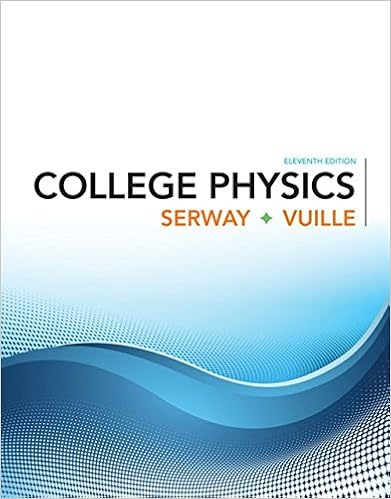# CHM 111 Practice Test 2 - CHEMISTRY 111 Practice Test 2 1...

• Test Prep
• 5
• 50% (2) 1 out of 2 people found this document helpful

This preview shows page 1 - 3 out of 5 pages.

CHM 111 Practice Test 2

1 .   How many moles of H + ( aq ) ions are present in 1.25 L of 0.75 M nitric acid ?
A. 0.60 mol
B. 0.75 mol
C. 0.94 mol
D. 1.7 mol
E. 1.9 mol
2 .   Which of the following will be least soluble in water ?
3 .   Select the precipitate that forms when the following reactants are mixed . Mg ( CH 3 COO ) 2 ( aq ) + LiOH ( aq ) 
4 .   Select the precipitate that forms when aqueous lead ( II ) nitrate reacts with aqueous sodium sulfate .
5 .   Consider the reaction : 3 Co 2 + ( aq ) + 6 NO 3 ¯ ( aq ) + 6 Na + ( aq ) + 2 PO 4 3 ¯ ( aq )  Co 3 ( PO 4 ) 2 ( s ) + 6 Na + ( aq ) + 6 NO 3 ¯ ( aq ) Identify the net ionic equation for this reaction .
A. Na + ( aq ) + NO 3 ¯ ( aq )  Na NO 3 ( aq )
B. 3 Co 2 + ( aq ) + NO 3 ¯ ( aq ) + Na + ( aq ) + 2 PO 4 3 ¯ ( aq )  Co 3 ( PO 4 ) 2 ( s ) + Na NO 3 ( aq )
C. 3 Co 2 + ( aq ) + 6 NO 3 ¯ ( aq ) + 6 Na + ( aq ) + 2 PO 4 3 ¯ ( aq )  Co 3 ( PO 4 ) 2 ( s ) + 6Na NO 3 ( aq )
D. 3 Co 2 + ( aq ) + 2 PO 4 3 ¯ ( aq )  Co 3 ( PO 4 ) 2 ( s )
E. None of these choices is correct .
Answer:  D.   3 Co 2 + ( aq ) + 2 PO 4 3 ¯ ( aq )  Co 3 ( PO 4 ) 2 ( s )
6 .   A base
7 .   Ammonia , NH 3 , produces hydroxide ions in aqueous solution by
8 .   Select the best statement relating to the following reaction : 2 MnO 2 ( s ) + KClO 3 ( aq ) + 2KOH ( aq )  2KMnO 4 ( aq ) + KCl ( aq ) + H 2 O ( l )
9 .   In a redox reaction , electrons are transferred
A. from the substance being oxidized to the reducing agent .
B. from the oxidizing agent to the reducing agent .
C. from the substance being reduced to the oxidizing agent .
D. from the substance being oxidized to the substance being reduced .
E. from the substance being reduced to the substance being oxidized .
Answer:  D )   from the substance being oxidized to the substance being reduced .
10 .   Calculate the oxidation number of sulfur in sodium metabisulfite , Na 2 S 2 O 5 .
11 .   The oxidation numbers of P , S and Cl in H 2 PO 2 ¯ , H 2 S and KClO 4 are , respectively
12 .   Select the classification for the following reaction . 2 Na ( s ) + 2H 2 O ( l )  2 NaOH ( aq ) + H 2 ( g )
13 .   A gas mixture consists of equal masses of methane ( molecular weight 16.0 ) and argon ( atomic weight 40.0 ) . If the partial pressure of argon is 200 . torr , what is the pressure of methane , in torr ?
A. 80.0 torr
B. 200 . torr
C. 256 torr
D. 500 . torr
E. 556 torr
Answer:  D )   500 . torr
14 .   A weather balloon was initially at a pressure of 0.950 atm , and it s volume was 35.0 L. The pressure decreased to 0.750 atm , without loss of gas or change in temperature . What was the change in the volume ?
15 .   A sample of nitrogen gas at 298 K and 745 torr has a volume of 37.42 L. What volume will it occupy if the pressure is increased to 894 torr at constant temperature ?
16 .   A carbon dioxide sample weighing 44.0 g occupies 32.68 L at 65  C and 645 torr . What is it s volume at STP ?
##### We have textbook solutions for you!
The document you are viewing contains questions related to this textbook.The document you are viewing contains questions related to this textbook.
Chapter 12 / Exercise 25
College Physics
Serway/VuilleExpert Verified
CHEMISTRY 111 Practice Test 21. How many moles of H+(aq) ions are present in 1.25 L of 0.75 Mnitric acid? A.0.60 molB.0.75 molC.0.94 molD.1.7 molE.1.9 mol
2.Which of the following will be least soluble in water?
3.Select the precipitate that forms when the following reactants are mixed.Mg(CH3COO)2(aq) + LiOH(aq)
4.Select the precipitate that forms when aqueous lead(II) nitrate reacts with aqueous sodium sulfate.
5.Consider the reaction:3Co2+(aq) + 6NO3¯(aq) + 6Na+(aq) + 2PO4(aq) Co3(PO4)2(s) + 6Na+(aq) + 6NO3¯(aq)Identify the net ionic equation for this reaction. A.Na+(aq) + NO3¯(aq) NaNO3(aqB.3Co2+(aq) + NO3¯(aq) + Na+(aq) + 2PO4(aq) Co3(PO4)2(s) + NaNO3(aqC.3Co2+(aq) + 6NO3¯(aq) + 6Na+(aq) + 2PO4(aq) Co3(PO4)2(s) + 6NaNO3(aqD.3Co2+(aq) + 2PO4(aq) Co3(PO4)2(sE.None of these choices is correct.
)
)
)
)
##### We have textbook solutions for you!
The document you are viewing contains questions related to this textbook.The document you are viewing contains questions related to this textbook.
Chapter 12 / Exercise 25
College Physics
Serway/VuilleExpert Verified
6.A base
•••